If I had to pick the single most important topic in software development, it would be data structures and algorithms. You can think of it as the fundamental tool available to every computer programmer. While programming, we use data structures to store and organize data, and algorithms to manipulate the data in those structures. This article contains a detailed review of all the common data structures and algorithms in Java to allow readers to become well equipped.

Listed below are the topics discussed in this article:

## Data Structures in Java

A data structure is a way of storing and organizing data in a computer so that it can be used efficiently. It provides a means to manage large amounts of data efficiently. And efficient data structures are key to designing efficient algorithms.

In this ‘Data Structures and Algorithms in Java’ article, we are going to cover basic data structures such as:

• Linear Data Structures
• Hierarchical Data Structures

Let’s check out each of them.

### Linear Data Structures in Java

Linear data structures in Java are those whose elements are sequential and ordered in a way so that: there is only one first element and has only one next element, there is only one last element and has only one previous element, while all other elements have a next and a previous element.

### Arrays

An array is a linear data structure representing a group of similar elements, accessed by index. Size of an array must be provided before storing data. Listed below are properties of an array:

• Each element in an array is of the same data type and has the same size
• Elements of the array are stored at contiguous memory locations with the first element is starting at the smallest memory location
• Elements of the array can be randomly accessed
• The array data structure is not completely dynamic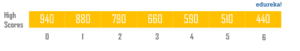For example, we may want a video game to keep track of the top ten scores for that game. Rather than use ten different variables for this task, we could use a single name for the entire group and use index numbers to refer to the high scores in that group.

### Linked List

A linked list is a linear data structure with the collection of multiple nodes, where each element stores its own data and a pointer to the location of the next element. The last link in a linked list points to null, indicating the end of the chain. An element in a linked list is called a node. The first node is called the head. The last node is called the tail.

Types of Linked List

Singly Linked List (Uni-Directional)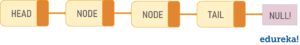Doubly Linked List (Bi-Directional)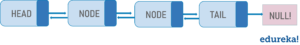Circular Linked List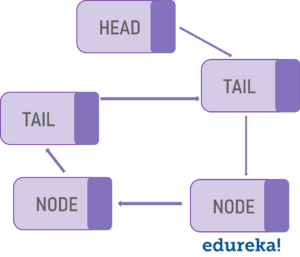Here’s a simple example: Imagine a linked list like a chain of paperclips that are linked together. You can easily add another paperclip to the top or bottom. It’s even quick to insert one in the middle. All you have to do is to just disconnect the chain at the middle, add the new paperclip, then reconnect the other half. A linked list is similar.

### Stacks

Stack, an abstract data structure, is a collection of objects that are inserted and removed according to the last-in-first-out (LIFO) principle. Objects can be inserted into a stack at any point of time, but only the most recently inserted (that is, “last”) object can be removed at any time. Listed below are properties of a stack: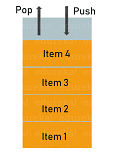• It is an orderd list in which insertion and deletion can be performed only at one end that is called the top
• Recursive data structure with a pointer to its top element
• Follows the last-in-first-out (LIFO) principle
• Supports two most fundamental methods
• push(e): Insert element e, to the top of the stack
• pop(): Remove and return the top element on the stack

Practical examples of the stack include when reversing a wordto check the correctness of parentheses sequence, implementing back functionality in browsers and many more.

### Queues

Queues are also another type of abstract data structure. Unlike a stack, the queue is a collection of objects that are inserted and removed according to the first-in-first-out (FIFO) principle. That is, elements can be inserted at any point of time, but only the element that has been in the queue the longest can be removed at any time. Listed below are properties of a queue: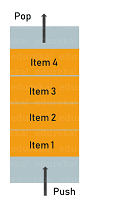• Often referred to as the first-in-first-out list
• Supports two most fundamental methods
• enqueue(e): Insert element e, at the rear of the queue
• dequeue(): Remove and return the element from the front of the queue

Queues are used in the asynchronous transfer of data between two processes, CPU scheduling, Disk Scheduling and other situations where resources are shared among multiple users and served on first come first server basis. Next up in this ‘Data Structures and Algorithms in Java’ article, we have hierarchical data structures.

### Binary Tree

Binary Tree is a hierarchical tree data structures in which each node has at most two children, which are referred to as the left child and the right child. Each binary tree has the following groups of nodes:

• Root Node: It is the topmost node and often referred to as the main node because all other nodes can be reached from the root
• Left Sub-Tree, which is also a binary tree
• Right Sub-Tree, which is also a binary tree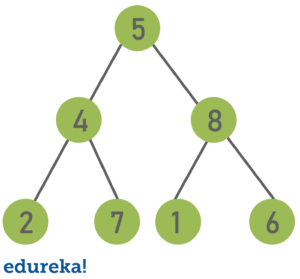Listed below are the properties of a binary tree:

• A binary tree can be traversed in two ways:
• Depth First Traversal: In-order (Left-Root-Right), Preorder (Root-Left-Right) and Postorder (Left-Right-Root)
• Breadth First Traversal: Level Order Traversal
• Time Complexity of Tree Traversal: O(n)
• The maximum number of nodes at level ‘l’ = 2l-1.

Applications of binary trees include:

• Used in many search applications where data is constantly entering/leaving
• As a workflow for compositing digital images for visual effects
• Used in almost every high-bandwidth router for storing router-tables
• Also used in wireless networking and memory allocation
• Used in compression algorithms and many more

### Binary Heap

Binary Heap is a complete binary tree, which answers to the heap property.  In simple terms it is a variation of a binary tree with the following properties:

• Heap is a complete binary tree: A tree is said to be complete if all its levels, except possibly the deepest, are complete. This property of Binary Heap makes it suitable to be stored in an array.
• Follows heap property: A Binary Heap is either a Min-Heap or a Max-Heap.
• Min Binary Heap: For every node in a heap, node’s value is lesser than or equal to values of the children
• Max Binary Heap: For every node in a heap, the node’s value is greater than or equal to values of the children

Popular applications of binary heap include implementing efficient priority-queues, efficiently finding the k smallest (or largest) elements in an array and many more.

### Hash Tables

Imagine that you have an object and you want to assign a key to it to make searching very easy. To store that key/value pair, you can use a simple array like a data structure where keys (integers) can be used directly as an index to store data values. However, in cases where the keys are too large and cannot be used directly as an index, a technique called hashing is used.

In hashing, the large keys are converted into small keys by using hash functions. The values are then stored in a data structure calledhash table. A hash table is a data structure that implements a dictionary ADT, a structure that can map unique keys to values.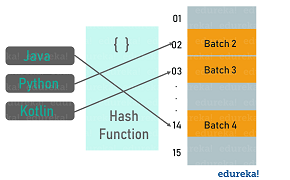In general, a hash table has two major components:

1. Bucket Array: A bucket array for a hash table is an array A of size N, where each cell of A is thought of as a “bucket”, that is, a collection of key-value pairs. The integer N defines the capacity of the array.
2. Hash Function: It is any function that maps each key k in our map to an integer in the range [0, N − 1], where N is the capacity of the bucket array for this table.

When we put objects into a hashtable, it is possible that different objects might have the same hashcode. This is called a collision. To deal with collision, there are techniques like chaining and open addressing.

So, these are some basic and most frequently used data structures in Java. Now that you are aware of each of these, you can start implementing them in your Java programs. With this, we have completed the first part of’ this ‘Data Structures and Algorithms in Java’ article. In the next part, we are going to learn about basic algorithms and how to use them in practical applications such as sorting and searching, divide and conquer, greedy algorithms, dynamic programming.

## Algorithms in Java

Historically used as a tool for solving complex mathematical computations, algorithms are deeply connected with computer science, and with data structures in particular. An algorithm is a sequence of instructions that describes a way of solving a specific problem in a finite period of time. They are represented in two ways:

• Flowcharts – It is a visual representation of an algorithm’s control flow
• Pseudocode – It is a textual representation of an algorithm that approximates the final source code

Note: The performance of the algorithm is measured based on time complexity and space complexity. Mostly, the complexity of any algorithm is dependent on the problem and on the algorithm itself.

Let’s explore the two major categories of algorithms in Java, which are:

### Sorting Algorithms in Java

Sorting algorithms are algorithms that put elements of a list in a certain order. The most commonly used orders are numerical order and lexicographical order. In this  ‘Data Structures and Algorithms’ article lets explore a few sorting algorithms.

Bubble Sort in Java

Bubble Sort, often referred to as sinking sort, is the simplest sorting algorithm. It repeatedly steps through the list to be sorted, compares each pair of adjacent elements and swaps them if they are in the wrong order. Bubble sort gets its name because it filters out the elements to the top of the array, like bubbles that float on water.

Here’s pseudocode representing Bubble Sort Algorithm (ascending sort context).

```a[] is an array of size N
begin BubbleSort(a[])

declare integer i, j
for i = 0 to N - 1
for j = 0 to N - i - 1
if a[j] > a[j+1] then
swap a[j], a[j+1]
end if
end for
return a

end BubbleSort
```

This code orders a one-dimensional array of N data items into ascending order. An outer loop makes N-1 passes over the array. Each pass uses an inner loop to exchange data items such that the next smallest data item “bubbles” towards the beginning of the array. But the problem is the algorithm needs one whole pass without any swap to know that the list is sorted.

Worst and Average Case Time Complexity: O(n*n). The worst-case occurs when an array is reverse sorted.

Best Case Time Complexity:O(n). Best case occurs when an array is already sorted.

### Selection Sort in Java

Selection sorting is a combination of both searching and sorting. The algorithm sorts an array by repeatedly finding the minimum element (considering ascending order) from the unsorted part and putting it at a proper position in the array.

Here’s pseudocode representing Selection Sort Algorithm (ascending sort context).

```a[] is an array of size N
begin SelectionSort(a[])

for i = 0 to n - 1
/* set current element as minimum*/
min = i
/* find the minimum element */
for j = i+1 to n
if list[j] < list[min] then
min = j;
end if
end for
/* swap the minimum element with the current element*/
if min != i  then
swap list[min], list[i]
end if
end for

end SelectionSort
```

As you can understand from the code, the number of times the sort passes through the array is one less than the number of items in the array. The inner loop finds the next smallest value and the outer loop places that value into its proper location. Selection sort never makes more than O(n) swaps and can be useful when the memory write is a costly operation.

Time Complexity: O(n2) as there are two nested loops.

Auxiliary Space: O(1).

### Insertion Sort in Java

Insertion Sort is a simple sorting algorithm which iterates through the list by consuming one input element at a time and builds the final sorted array. It is very simple and more effective on smaller data sets. It is stable and in-place sorting technique.

Here’s pseudocode representing Insertion Sort Algorithm (ascending sort context).

```a[] is an array of size N
begin InsertionSort(a[])

for i = 1 to N
key = a[ i ]
j = i - 1
while ( j >= 0 and a[ j ] > key0
a[ j+1 ] = x[ j ]
j = j - 1
end while
a[ j+1 ] = key
end for

end InsertionSort
```

As you can understand from the code, the insertion sort algorithm removes one element from the input data, finds the location it belongs within the sorted list and inserts it there. It repeats until no input elements remain unsorted.

Best Case: The best case is when input is an array that is already sorted. In this case insertion sort has a linear running time (i.e., Θ(n)).

Worst Case: The simplest worst case input is an array sorted in reverse order.

### QuickSort in Java

Quicksort algorithm is a fast, recursive, non-stable sort algorithm which works by the divide and conquer principle. It picks an element as pivot and partitions the given array around that picked pivot.

Steps to implement Quick sort:

1. Pick a suitable “pivot point”.
2. Divide the lists into two lists based on this pivot element. Every element which is smaller than the pivot element is placed in the left list and every element which is larger is placed in the right list. If an element is equal to the pivot element then it can go in any list. This is called the partition operation.
3. Recursively sort each of the smaller lists.

Here’s pseudocode representing Quicksort Algorithm.

```QuickSort(A as array, low as int, high as int){
if (low < high){
pivot_location = Partition(A,low,high)
Quicksort(A,low, pivot_location)
Quicksort(A, pivot_location + 1, high)
}
}
Partition(A as array, low as int, high as int){
pivot = A[low]
left = low

for i = low + 1 to high{
if (A[i] < pivot) then{
swap(A[i], A[left + 1])
left = left + 1
}
}
swap(pivot,A[left])

return (left)}
```

In the above pseudocode, partition() function performs partition operation and Quicksort() function repeatedly calls partition function for each smaller list generated. The complexity of quicksort in the average case is Θ(n log(n)) and in the worst case is Θ(n2).

### Merge Sort in Java

Mergesort is a fast, recursive, stable sort algorithm which also works by the divide and conquer principle. Similar to quicksort, merge sort divides the list of elements into two lists. These lists are sorted independently and then combined. During the combination of the lists, the elements are inserted (or merged) at the right place in the list.

Here’s pseudocode representing Merge Sort Algorithm.

```procedure MergeSort( a as array )
if ( n == 1 ) return a

var l1 as array = a ... a[n/2]
var l2 as array = a[n/2+1] ... a[n]

l1 = mergesort( l1 )
l2 = mergesort( l2 )

return merge( l1, l2 )
end procedure

procedure merge( a as array, b as array )

var c as array
while ( a and b have elements )
if ( a > b )
add b to the end of c
remove b from b
else
add a to the end of c
remove a from a
end if
end while

while ( a has elements )
add a to the end of c
remove a from a
end while

while ( b has elements )
add b to the end of c
remove b from b
end while

return c

end procedure
```

mergesort() function divides the list into two, calls mergesort() on these lists separately and then combines them by sending them as parameters to merge() function. The algorithm has a complexity of O(n log (n)) and has a wide range of applications.

### Heap Sort in Java

Heapsort is a comparison-based sorting algorithm Binary Heap data structure. You can think of it as improved version f selection sort, where it divides its input into a sorted and an unsorted region, and it iteratively shrinks the unsorted region by extracting the largest element and moving that to the sorted region.

Steps to implement Quicksort(in increasing order):

1. Build a max heap with the sorting array
2. At this point, the largest item is stored at the root of the heap. Replace it with the last item of the heap and reduce the size of heap by 1. Finally, heapify the root of tree
3. Repeat the above steps until the size of the heap is greater than 1

Here’s pseudocode representing Heap Sort Algorithm.

```Heapsort(a as array)

for (i = n / 2 - 1) to i >= 0
heapify(a, n, i);

for i = n-1 to 0
swap(a, a[i])
heapify(a, i, 0);
end for
end for

heapify(a as array, n as int, i as int)
largest = i //Initialize largest as root
int l eft = 2*i + 1; // left = 2*i + 1
int right = 2*i + 2; // right = 2*i + 2

if (left < n) and (a[left] > a[largest])
largest = left

if (right < n) and (a[right] > a[largest])
largest = right

if (largest != i)
swap(a[i], A[largest])
Heapify(a, n, largest)
end heapify
```

Apart from these, there are other sorting algorithms which are not that well known such as, Introsort, Counting Sort, etc. Moving on to the next set of algorithms in this ‘Data Structures and Algorithms’ article, let’s explore searching algorithms.

### Searching Algorithms in Java

Searching is one of the most common and frequently performed actions in regular business applications. Search algorithms are algorithms for finding an item with specified properties among a collection of items. Let’s explore two of the most commonly used searching algorithms.

### Linear Search Algorithm in Java

Linear search or sequential search is the simplest search algorithm. It involves sequential searching for an element in the given data structure until either the element is found or the end of the structure is reached. If the element is found, then the location of the item is returned otherwise the algorithm returns NULL.

Here’s pseudocode representing Linear Search in Java:

```procedure linear_search (a[] , value)
for i = 0 to n-1
if a[i] = value then
print "Found "
return i
end if
print "Not found"
end for

end linear_search```

It is a brute-force algorithm. While it certainly is the simplest, it’s most definitely is not the most common, due to its inefficiency. Time Complexity of Linear search is O(N).

### Binary Search Algorithm in Java

Binary search, also known as logarithmic search, is a search algorithm that finds the position of a target value within an already sorted array. It divides the input collection into equal halves and the item is compared with the middle element of the list. If the element is found, the search ends there. Else, we continue looking for the element by dividing and selecting the appropriate partition of the array, based on if the target element is smaller or bigger than the middle element.

Here’s pseudocode representing Binary Search in Java:

```Procedure binary_search
a; sorted array
n; size of array
x; value to be searched

lowerBound = 1
upperBound = n

while x not found
if upperBound < lowerBound
EXIT: x does not exists.

set midPoint = lowerBound + ( upperBound - lowerBound ) / 2

if A[midPoint] < x set lowerBound = midPoint + 1 if A[midPoint] > x
set upperBound = midPoint - 1

if A[midPoint] = x
EXIT: x found at location midPoint
end while

end procedure```

The search terminates when the upperBound (our pointer) goes past lowerBound (last element), which implies we have searched the whole array and the element is not present. It is the most commonly used search algorithms primarily due to its quick search time. The time complexity of the binary search is O(N) which is a marked improvement on the O(N) time complexity of Linear Search.

This brings us to the end of this ‘Data Structures and Algorithms in Java’ article. I have covered one of the most fundamental and important topics of Java. Hope you are clear with all that has been shared with you in this article.

Make sure you practice as much as possible and revert your experience.

Check out the Java Training by Edureka, a trusted online learning company with a network of more than 250,000 satisfied learners spread across the globe. We are here to help you with every step on your journey, for becoming a besides this java interview questions, we come up with a curriculum which is designed for students and professionals who want to be a Java Developer.

Got a question for us? Please mention it in the comments section of this ‘Data Structures and Algorithms in Java’ article and we will get back to you as soon as possible.